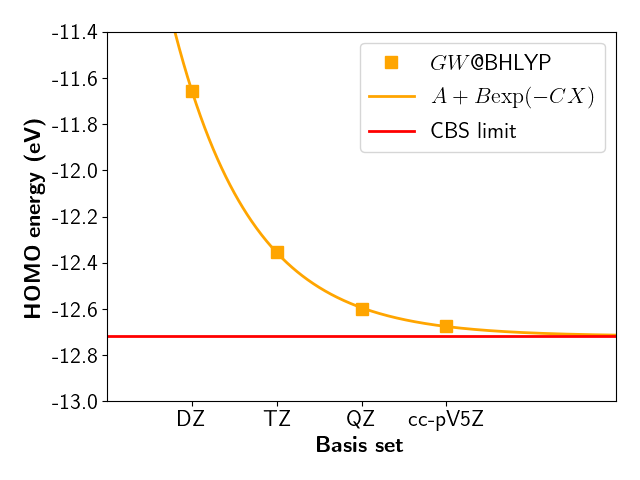# GW with fully analytic formula (general)

Here follows the most standard $$GW$$ calculation with MOLGW for the ionization potential (IP) of water. $$G_0W_0$$ based on BHLYP (50 %) of exact-exchange is known to be very good for IPs.

&molgw
comment='H2O GW analytic formula'    ! an optional plain text here

scf='BHLYP'

basis='cc-pVTZ'
auxil_basis='cc-pVTZ-RI'

postscf='G0W0'

selfenergy_state_range=0      ! will calculate just the HOMO
frozencore='yes'              ! accurate approximation: O1s will not be included
! in the RPA/GW calculation

natom=3
/
O      0.000000  0.000000  0.119262
H      0.000000  0.763239 -0.477047
H      0.000000 -0.763239 -0.477047


After the SCF cycles with the BHLYP hybrid functinal, one can find the response calculation within the RPA equation:

 Prepare a polarizability spectral function with
Occupied states:        4
Virtual states:       53
Transition space:      212


MOLGW will then diagonalize a $$212 \times 212$$ matrix. This is must often the computational bottleneck in a calculation. Memory scales as $$N^4$$ and computer time as $$N^6$$.

Then comes the $$GW$$ quasiparticle energies and weights:

 state spin    QP energy (eV)  QP spectral weight
5    1     -12.354761       0.915241


State 5 is the HOMO. The $$GW$$ ionization potential is the negative HOMO, here 12.35 eV.

Experimental value is 12.62 eV

The discrepancy is large. Why?

## GW slow convergence

A complete basis convergnce study for GW@BHLYP would givewith a CBS HOMO evaluated to -12.72 eV.

MOLGW automatically proposes a CBS extrapolated value based a trained linear regression:

 Extrapolation to CBS (eV)
<i|-\nabla^2/2|i>    Delta E_i     E_i(cc-pVTZ)      E_i(CBS)
state    5 spin  1 :        62.271159       -0.404451      -12.354761      -12.759212

where the $$GW$$ HOMO energy is -12.76 eV. So 0.04 eV away from the correct CBS, but the raw cc-pVTZ value was 0.37 eV away.

Now the experimental and $$GW$$ IP agree within 0.14 eV. This is the typical accuracy of $$GW$$ calculations.

# GW with imaginary frequencies (HOMO LUMO gap)

If we are just interested in the HOMO LUMO region, there exists an alternative to the fully diagonalization of the RPA equation ($$N^6$$ scaling).

The $$GW$$ self-energy can be evaluated by numerical quadruture for imaginary frequencies and then analytically continued to real frequencies with a Padé approximant.

This is much faster ($$N^4$$ scaling), however it is robust only in the HOMO-LUMO gap region and it requires more convergence parameters.

Here is a typical input file:

&molgw
comment='H2O GW with numerical integration'

scf='BHLYP'

basis='cc-pVTZ'
auxil_basis='cc-pVTZ-RI'

postscf='G0W0_PADE'

selfenergy_state_range=0
frozencore='yes'

nomega_chi_imag=16           ! frequency grid for the response function
nomega_sigma_calc=12         ! frequency grid for sigma along the imaginary axis
! (fit of the analytic continuation)
step_sigma_calc=0.1
nomega_sigma=101             ! frequency grid for sigma along the real axis

natom=3
/
O      0.000000  0.000000  0.119262
H      0.000000  0.763239 -0.477047
H      0.000000 -0.763239 -0.477047


The output reports a HOMO value of -12.354 eV, which agrees within 1 meV with the previous analytic value.

 state spin    QP energy (eV)  QP spectral weight
5    1     -12.354356       0.915308